Get instant live expert help with Excel or Google Sheets“My Excelchat expert helped me in less than 20 minutes, saving me what would have been 5 hours of work!”

#### Post your problem and you’ll get expert help in seconds.

Your message must be at least 40 characters
Our professional experts are available now. Your privacy is guaranteed.

# How to Use the DATA VALIDATION ISNUMBER Function in Excel

You can create your own data validation to allow numbers only in Excel. To do this, you need to use the ISNUMBER function. In this tutorial, you will learn how to create a data validation to allow only allow numbers using the ISNUMBER function.

## Use data validation in Excel to only allow numbers

### How to use the ISNUMBER Function in Excel for Data Validation

Data validation is a good practice to reduce issues during an audit. The data validation ISNUMBER function helps maintain this by allowing only number and date values. In the following example, you have two columns of values and results. The values column has data belonging to different types, and the result column shows whether the values are numbers. To do this:

• Go to cell B2.
• Insert the formula `=IF(ISNUMBER(B2),”Number”,""). `
• Click Enter
• Drag the formula to the cells B2 to B8 with your mouse.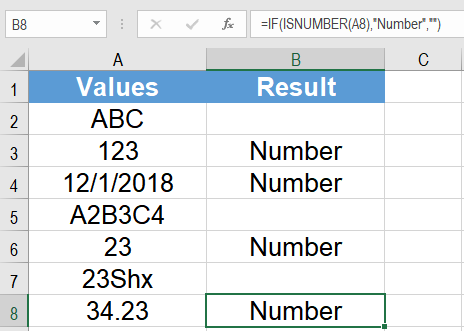This will show in column B whether the values in column A are numbers.

### Data Validation to Allow Numbers only

Using this logic, you can set a data validation rule that allows only numbers in a column. The following example uses a student data sheet. Column A has the names, column B has the classes, and Column C will have the ages. To limit column C to accept only number values:

1. Select cells C2:C6 by selecting cell C2 and dragging it till cell C6 with your mouse.
2. Go to Data > Data Validation.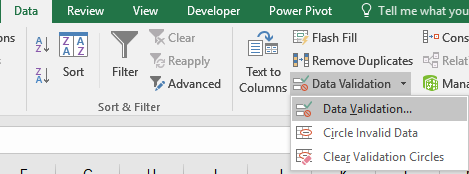3. Set Allow to Custom.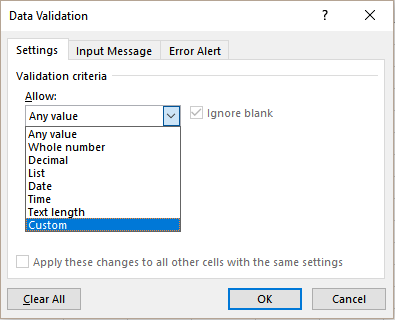4. In the Formula field type the formula `=ISNUMBER(C2)`.
5. Click Ok.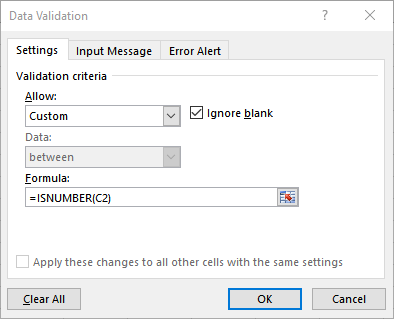This will set a data validation to allow numbers only in column C. Trying other data types will result in an error. You can set your own custom error message. To do this:

• After completing step 4, go to the Error Alert tab and set the Error Message to“Input not a number. Please enter a valid number.”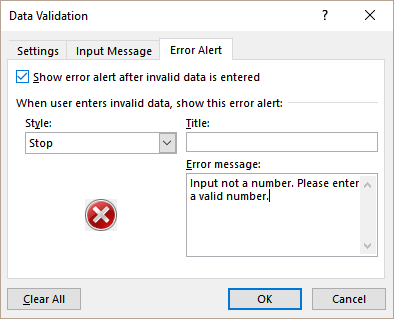• Click Ok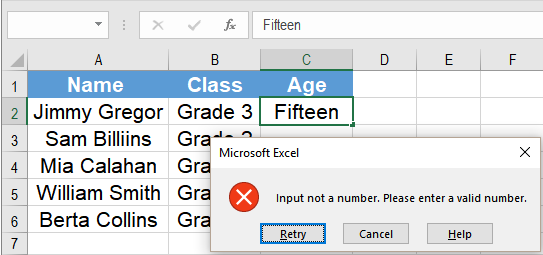If you try to enter a non-number in column C, Excel will show this message now.

Still need some help with Excel formatting or have other questions about Excel? Connect with a live Excel expert here for some 1 on 1 help. Your first session is always free.

### Did this post not answer your question? Get a solution from connecting with the expert.Another blog reader asked this question today on Excelchat:
Related blogs
Solution examplesI need a formula to count cells with a value containing the word "arc" or the word "robot". The cells are all in the same column, but some of the cells contain both words so I get duplicates in my count.
Solved by M. W. in 39 minsI have a main dataset of about 500,000 line items. I want to lookup the items from 3 different datasets. Each of these datasets has got about 200,000 line items. I want to use the if and vlookup function to return a " Yes" or "No" answer.
Solved by O. Q. in 40 minsI have a column where each cell has letters and numbers. The numbers would range from 10 through 18. I would like to find a formula where I can search the cell for any one of the numbers and return a value from a different cell if it finds one. I currently search for a specific number and the formula works but only for that particular number. The formula I currently have is: =IF(ISNUMBER(SEARCH("16",F10)),G10,"") This does work if it finds the value of 16 in the (F10)cell and then places the value from cell "G10" as the result. If it doesn't find the numbers, it will leave the resultant cell blank.
Solved by O. H. in 60 minsI need to modify my formula below to automatically the change cell color to yellow if the result in result in cell H3 is greater than 30. This formula is in cell H3 =IF(ISNUMBER(B3), TODAY()-B3, " ")
Solved by O. J. in 39 minsI want to display a range of cells if they meet a specific criteria. I want to display a name IF it does not equal one of three options AND there are any numbers contained in a range of cells
Solved by G. F. in 60 mins## Subscribe to Excelchat.coAnother blog reader asked this question today on Excelchat: